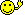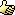CodeGuru Home VC++ / MFC / C++ .NET / C# Visual Basic VB Forums Developer.com

# Thread: Build this simple progam according to earlier standards

1.MemberJoin Date
Jul 2007
Posts
54

## Build this simple progam according to earlier standards

I am new to C++ and have learned that when I build a program and I want to learn how, it is best to build each program according to the same standard.

In my programs I want to have a function protocol before the main section and a function definition after the main section.

Now I would like to build a program that takes 2 integers and switches them, integer A becomes integer B and the other way around. Simple.

What I have now is this:

Code:
```#include <iostream>
using namespace std;

int ChangeInteger(int, int, int); // protocol

int main()
{
int IntegerA;
int IntegerB;
int IntegerTemp;

cout << "Type 1st integer: ";
cin  >> IntegerA;

cout << "Type 2nd integer: ";
cin  >> IntegerB;

IntegerTemp = 5;

cout << "The value of IntegerA is " << ChangeInteger(IntegerA, IntegerB, IntegerTemp) << endl;

system ("pause");

return 0;
}

int ChangeInteger (int Integer_A, int Integer_B, int Integer_Temp) // definition

{
int result = Integer_B;
return result;
}

int ChangeInteger2(int, int, int); // protocol

{
int IntegerA;
int IntegerB;
int IntegerTemp;

cout << "The value of IntegerB is " << ChangeInteger2(IntegerA, IntegerB, IntegerTemp) << endl;

int ChangeInteger2 (int Integer_A, int Integer_B, int Integer_Temp) // definition

int result2 = Integer_A;
return result2;
}```
This is not working, it is giving me the following error on the "{" sign that comes below the line with the "int ChangeInteger2 (int Integer_A, int Integer_B, int Integer_Temp)" function definition.

error C2447: '{' : missing function header (old-style formal list?)

By the way, is it good practice to have a protocol and a definition in each program?Reply With Quote

2.Elite Member Power PosterJoin Date
Jan 2006
Location
Singapore
Posts
6,768

## Re: Build this simple progam according to earlier standards

It is good to use code tags, but better to indent your code so that the code tags become effective.

This is not working, it is giving me the following error on the "{" sign that comes below the line with the "int ChangeInteger2 (int Integer_A, int Integer_B, int Integer_Temp)" function definition.
ChangeInteger2 looks problematic in that the line with the "definition" comment is out of place. It looks as if you are trying to redefine the function in its body.

By the way, is it good practice to have a protocol and a definition in each program?
I think you mean prototype and definition. I would say that this is usually good practice. The prototypes (and class declarations) would go in header files, and the function and class implementation into the source files.

What you are trying to do is to swap two variables. Typically we do use a temporary variable to perform the swap, but this variable will be local to the swap function. Instead of returning a value (you cannot really swap just by returning a value), we would pass the variables as references. An example (implementation not shown) would be:
Code:
```void swap(int& a, int& b)
{
// Create a temporary int.
// Use the temporary to perform the swap.
}```
Note that the standard library provides a generic std::swap with the same function signature, so my example might not work since you are using namespace std.Reply With Quote

3. ## Re: Build this simple progam according to earlier standards

Looks like LaserLight caught most of the problems with your code.Just wanted to point out that there is NO need for a temporary variable to swap integers...

Code:
```void Swap( int &a, int &b)
{
a = a ^ b;
b = b ^ a;
a = a ^ b;
}```
Even if you dont want to use this technique, there is still no reason to PASS the temporary...

Code:
```void Swap( int &a, int &b)
{
int tmp = a;
a = b;
b = tmp;
}```Reply With Quote

4.MemberJoin Date
Aug 2005
Location
India
Posts
67

## Re: Build this simple progam according to earlier standards

Code:
```int ChangeInteger2(int, int, int); // protocol

{
int IntegerA;
int IntegerB;
int IntegerTemp;

cout << "The value of IntegerB is " << ChangeInteger2(IntegerA, IntegerB, IntegerTemp) << endl;

int ChangeInteger2 (int Integer_A, int Integer_B, int Integer_Temp) // definition

int result2 = Integer_A;
return result2;
}```
If you need to define function ChangeInteger2(); then define it as u have defined ChangeInteger();

"int ChangeInteger2(int, int, int); // protocol" is your declaration. Declaring a function before any function just says u will use the declared function before its defination.

And search a better logic to "swap two variables".
Last edited by Mavens; July 22nd, 2007 at 11:35 AM. Reason: Code tags missedReply With Quote

5.Elite Member Power PosterJoin Date
Jan 2006
Location
Singapore
Posts
6,768

## Re: Build this simple progam according to earlier standards

Just wanted to point out that there is NO need for a temporary variable to swap integers...
Note however that this pertains to integers. The use of a temporary is more typical and generic.

Even if you dont want to use this technique, there is still no reason to PASS the temporary...
Tsk tsk, people give out Harry Potter spoilers but you give out swap code spoilersReply With Quote

6.MemberJoin Date
Jul 2007
Posts
54

## Re: Build this simple progam according to earlier standardsOriginally Posted by Mavens
Code:
```int ChangeInteger2(int, int, int); // protocol

{
int IntegerA;
int IntegerB;
int IntegerTemp;

cout << "The value of IntegerB is " << ChangeInteger2(IntegerA, IntegerB, IntegerTemp) << endl;

int ChangeInteger2 (int Integer_A, int Integer_B, int Integer_Temp) // definition

int result2 = Integer_A;
return result2;
}```
If you need to define function ChangeInteger2(); then define it as u have defined ChangeInteger();

"int ChangeInteger2(int, int, int); // protocol" is your declaration. Declaring a function before any function just says u will use the declared function before its defination.

And search a better logic to "swap two variables".
What do you mean exactly, it looks to me as if I defined them identically allready.

Also, could somebody explain to me how this works:

Code:
```int tmp = a;
a = b;
b = tmp;```
Thanks!
Last edited by Break2; July 22nd, 2007 at 12:47 PM.Reply With Quote

7.MemberJoin Date
Aug 2005
Location
India
Posts
67

## Re: Build this simple progam according to earlier standards

You havnt defined that identically.

A function defination contains
return type function_name(parameters)
{
Internal defination
return value
}

what you have done is
function_name(parameters); // See a semicolon there
{
some Internal defination. // useless stuff.
started a function defination again. // It seems that u dont know how to define and use a function.
}

Explanation of

int tmp = a; // You have taken a temporary variable in which u put value
of a.
a = b; // Now put value of b in a.
b = tmp; // and finally put value of tmp in a.

So you hav got value of a and b in b and a respectively. which is called swapping of two variables.Reply With Quote

8.MemberJoin Date
Jul 2007
Posts
54

## Re: Build this simple progam according to earlier standardsOriginally Posted by Mavens
It seems that u dont know how to define and use a function
I think you hit the nail right on the spot there. Sometimes I do not know exactly what I am doing when it comes to defining and using a function.

Some of the absolute noob questions I have are:

- Do I always need to use a prototype before the 'main' section?
- I like to keep the prototype and definition seperated, prototype above the main section, definition below main, is this always applicable and good practice?
- When do I have to use "return 0" and when do I return an actual value?
- Isn't the left part of the function actually a variable?
If I for instance have:
Code:
`char x = (Character_1 + 1)`
Isnt it so that "char x = (Character_1 + 1)" is a function while "char x" is also a variable?

Yes, yes, I know, absolute noob questions.
Last edited by Break2; July 23rd, 2007 at 04:28 AM.Reply With Quote

9.MemberJoin Date
Jan 2007
Posts
94

## Re: Build this simple progam according to earlier standardsOriginally Posted by Break2
- Do I always need to use a prototype before the 'main' section?
Not always, however, it is a good habit to always use prototypes.

- I like to keep the prototype and definition seperated, prototype above the main section, definition below main, is this always applicable and good practice?

- When do I have to use "return 0" and when do I return an actual value?
That depends entirely on the code you're writing. there's nothing especially meaningful about zero, its just a number. But depending on the context of the function, it can be very useful.

More useful, possibly, in 'C' style code. Much of the C standard library uses functions which 'return 0;' for various reasons - probably because C didn't have a built in 'bool' type. in C++ i find it less useful, since most situations simply require a true/false value.

return 0 sometimes works well in conjunction with pointers, although if you're just getting to grips with functions you shouldn't be worrying about pointers for a while yet

- Isn't the left part of the function actually a variable?
If I for instance have:
Code:
`char x = (Character_1 + 1)`
Isnt it so that "char x = (Character_1 + 1)" is a function while "char x" is also a variable?
No, this is a statement containing an expression. 'x' is a char variable - which is assigned the result of the expression Character_1 + 1

a function is a named block of code. If you wanted to write a function to perform the above expression, it could look something like this
Code:
```char my_function(char);    //Function Prototype

int main()
{
char my_character = 'C';
char x = my_function( my_character );  //Statement with a function call
}

//Here is the function itself
char my_function(char Character_1)
{
return Character_1 + 1;
}```
Yes, yes, I know, absolute noob questions.
Nothing wrong with asking questions, we were all beginners onceI do suggest you read a good tutorial or book which thoroughly explains functions though.
Last edited by Bench_; July 23rd, 2007 at 08:48 AM.Reply With Quote

####Posting Permissions

• You may not post new threads
• You may not post replies
• You may not post attachments
• You may not edit your posts
•